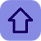# How We Can Have a Nested Return In a Function

Posted by Tushar Bedekar
Let us First Understand what is required and what the question is all about.Actually we are discussing about nested return in the function. that is return within the return  so follow is the programme which is conveying the same.Her we have taken the two function name "Factorial" and "Sum" which are calculating the factorial of a number and the result of the factorial  is returned to the function name sum which is calculating the sum of any number

#include <stdio.h>
#include <stdlib.h>

int factorial();
int sum();

int main(int a)
{
int c;
printf("\"enter the a number\"");
scanf("%d",&a);

c=sum(a);
printf("The required result is %d \n",c);
getchar();return 0;
}

int factorial(int y)
{
int i,fact=1;
for(i=1;i<=y;i++)
fact=fact*i;
printf("The factorial of a number is %d \n",fact);
return fact;
}

int sum( int x)
{
int result,k;
printf("enter the number whose factorial is required \n");
scanf("%d",&k);
result=x+factorial(k);
return result;
}